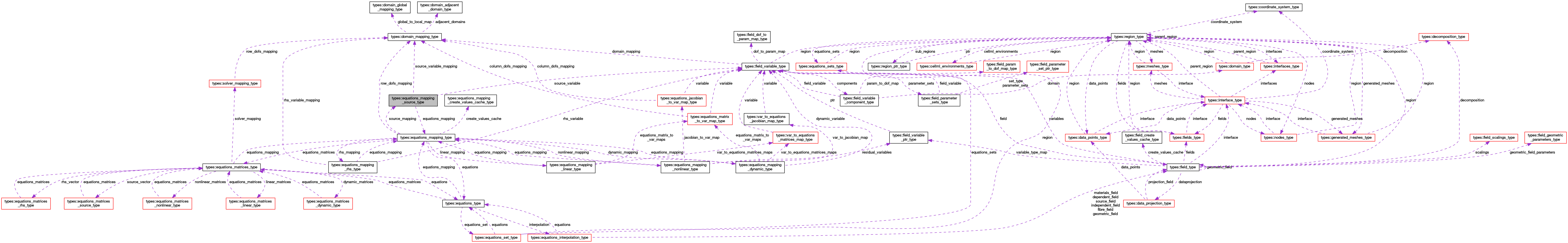OpenCMISS-Iron Internal API Documentation
types::equations_mapping_source_type Type Reference

Contains information on the equations mapping for a source i.e., how a field variable is mapped to the source vector for the equations set of this equations mapping. More...

Collaboration diagram for types::equations_mapping_source_type:[legend]

## Public Attributes

type(equations_mapping_type), pointer equations_mapping
A pointer to the equations mapping. More...

integer(intg) source_variable_type
The variable type number mapped from the source vector. More...

type(field_variable_type), pointer source_variable
A pointer to the source variable. More...

type(domain_mapping_type), pointer source_variable_mapping
A pointer to the domain mapping for the source variable. More...

real(dp) source_coefficient
The multiplicative coefficient applied to the source vector. More...

integer(intg), dimension(:), allocatable source_dof_to_equations_row_map
SOURCE_DOF_TO_EQUATIONS_ROW_MAP(source_dof_idx). The mapping from the source_dof_idx'th source dof in the source variable to the equations row. More...

integer(intg), dimension(:), allocatable equations_row_to_source_dof_map
EQUATIONS_ROW_TO_SOURCE_DOF_MAP(row_idx). The mapping from the row_idx'th row of the equations to the source dof. More...

## Detailed Description

Contains information on the equations mapping for a source i.e., how a field variable is mapped to the source vector for the equations set of this equations mapping.

Definition at line 1647 of file types.f90.

## Member Data Documentation

 type(equations_mapping_type), pointer types::equations_mapping_source_type::equations_mapping

A pointer to the equations mapping.

Definition at line 1648 of file types.f90.

 integer(intg), dimension(:), allocatable types::equations_mapping_source_type::equations_row_to_source_dof_map

EQUATIONS_ROW_TO_SOURCE_DOF_MAP(row_idx). The mapping from the row_idx'th row of the equations to the source dof.

Definition at line 1654 of file types.f90.

 real(dp) types::equations_mapping_source_type::source_coefficient

The multiplicative coefficient applied to the source vector.

Definition at line 1652 of file types.f90.

 integer(intg), dimension(:), allocatable types::equations_mapping_source_type::source_dof_to_equations_row_map

SOURCE_DOF_TO_EQUATIONS_ROW_MAP(source_dof_idx). The mapping from the source_dof_idx'th source dof in the source variable to the equations row.

Definition at line 1653 of file types.f90.

 type(field_variable_type), pointer types::equations_mapping_source_type::source_variable

A pointer to the source variable.

Definition at line 1650 of file types.f90.

 type(domain_mapping_type), pointer types::equations_mapping_source_type::source_variable_mapping

A pointer to the domain mapping for the source variable.

Definition at line 1651 of file types.f90.

 integer(intg) types::equations_mapping_source_type::source_variable_type

The variable type number mapped from the source vector.

Definition at line 1649 of file types.f90.